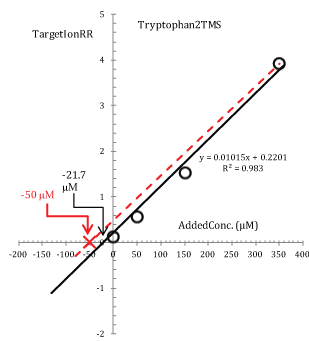Figure 7: Modified standard additions plot for Tryptophan2TMS (target ion 202.15 Th; data from the main basic experiment in Figure 1). Experimental points are interpolated with a least square regression line whose equation is given in the plot (solid line). The broken line is drawn through the experimental point at an added concentration of 350 μM and is forced to intercept the abscissa -50 μM in order to stress inconsistency between points within the calibration curve.